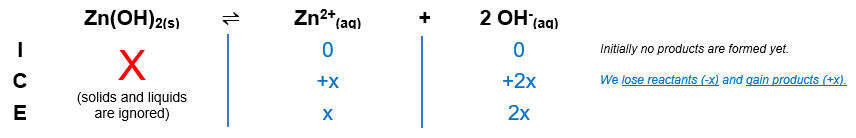# Problem: What is the pH of a saturated solution of Zn(OH)2? Ksp=1.8x10-14

###### FREE Expert Solution

Zn(OH)2(s)  Zn2+(aq) + 2 OH(aq)The Ksp expression for Zn(OH)2 is:

$\overline{){{\mathbf{K}}}_{{\mathbf{sp}}}{\mathbf{=}}\frac{\mathbf{products}}{\overline{)\mathbf{reactants}}}{\mathbf{=}}{\mathbf{\left[}}{{\mathbf{Zn}}}^{\mathbf{2}\mathbf{+}}{\mathbf{\right]}}{\mathbf{\left[}}{{\mathbf{OH}}}^{{\mathbf{-}}}{{\mathbf{\right]}}}^{{\mathbf{2}}}}$

Calculate OH- concentration:

${\mathbf{K}}_{\mathbf{sp}}\mathbf{=}\left(x\right){\left(2x\right)}^{\mathbf{2}}\phantom{\rule{0ex}{0ex}}{\mathbf{K}}_{\mathbf{sp}}\mathbf{=}\mathbf{x}\left(4{x}^{2}\right)$

${\mathbf{K}}_{\mathbf{sp}}\mathbf{=}\mathbf{4}{\mathbf{x}}^{\mathbf{3}}$

$\frac{\mathbf{1}\mathbf{.}\mathbf{8}\mathbf{×}{\mathbf{10}}^{\mathbf{-}\mathbf{14}}}{\mathbf{4}}\mathbf{=}\frac{\overline{)\mathbf{4}}{\mathbf{x}}^{\mathbf{3}}}{\overline{)\mathbf{4}}}\phantom{\rule{0ex}{0ex}}\sqrt[\mathbf{3}]{\mathbf{4}\mathbf{.}\mathbf{5}\mathbf{×}{\mathbf{10}}^{\mathbf{-}\mathbf{15}}}\mathbf{=}\sqrt[\mathbf{3}]{{\mathbf{x}}^{\mathbf{3}}}$

x = 1.651 × 10–5 M

97% (199 ratings)###### Problem Details

What is the pH of a saturated solution of Zn(OH)2? Ksp=1.8x10-14